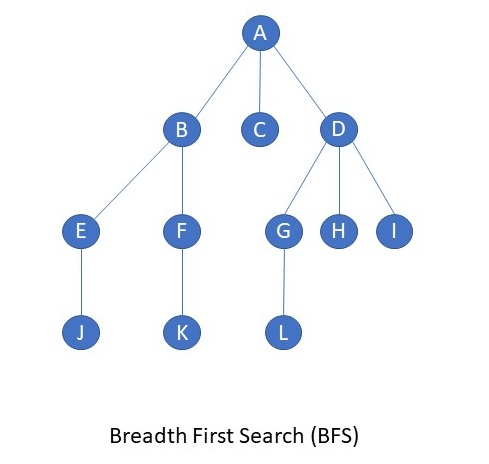# Breadth First Search (BFS) in C

C program to implement Breadth First Search(BFS). Breadth First Search is an algorithm used to search a Tree or Graph. BFS search starts from root node then traverses into next level of graph or tree, if item found it stops other wise it continues with other nodes in the same level before moving on to the next level. The algorithm can also be used for just Tree/Graph traversal, without actually searching for a value. This is what being done in the program below. The disadvantage of BFS is it requires more memory compare to Depth First Search(DFS).### The Program

This file contains bidirectional Unicode text that may be interpreted or compiled differently than what appears below. To review, open the file in an editor that reveals hidden Unicode characters. Learn more about bidirectional Unicode characters
 #include int a, q, visited, n, i, j, f = 0, r = -1; void bfs(int v) { for(i = 1; i <= n; i++) if(a[v][i] && !visited[i]) q[++r] = i; if(f <= r) { visited[q[f]] = 1; bfs(q[f++]); } } void main() { int v; printf("\n Enter the number of vertices:"); scanf("%d", &n); for(i=1; i <= n; i++) { q[i] = 0; visited[i] = 0; } printf("\n Enter graph data in matrix form:\n"); for(i=1; i<=n; i++) { for(j=1;j<=n;j++) { scanf("%d", &a[i][j]); } } printf("\n Enter the starting vertex:"); scanf("%d", &v); bfs(v); printf("\n The node which are reachable are:\n"); for(i=1; i <= n; i++) { if(visited[i]) printf("%d\t", i); else { printf("\n Bfs is not possible. Not all nodes are reachable"); break; } } }
view raw bfs.c hosted with ❤ by GitHub